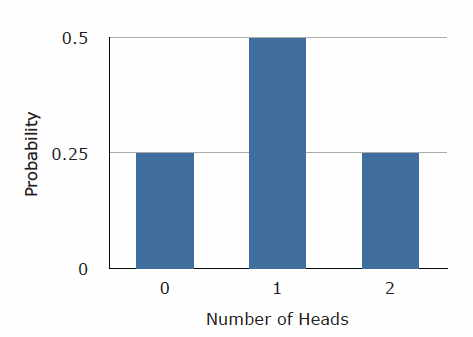# 5.7: Binomial Distribution

•• David Lane
• Rice University
$$\newcommand{\vecs}{\overset { \rightharpoonup} {\mathbf{#1}} }$$ $$\newcommand{\vecd}{\overset{-\!-\!\rightharpoonup}{\vphantom{a}\smash {#1}}}$$$$\newcommand{\id}{\mathrm{id}}$$ $$\newcommand{\Span}{\mathrm{span}}$$ $$\newcommand{\kernel}{\mathrm{null}\,}$$ $$\newcommand{\range}{\mathrm{range}\,}$$ $$\newcommand{\RealPart}{\mathrm{Re}}$$ $$\newcommand{\ImaginaryPart}{\mathrm{Im}}$$ $$\newcommand{\Argument}{\mathrm{Arg}}$$ $$\newcommand{\norm}{\| #1 \|}$$ $$\newcommand{\inner}{\langle #1, #2 \rangle}$$ $$\newcommand{\Span}{\mathrm{span}}$$ $$\newcommand{\id}{\mathrm{id}}$$ $$\newcommand{\Span}{\mathrm{span}}$$ $$\newcommand{\kernel}{\mathrm{null}\,}$$ $$\newcommand{\range}{\mathrm{range}\,}$$ $$\newcommand{\RealPart}{\mathrm{Re}}$$ $$\newcommand{\ImaginaryPart}{\mathrm{Im}}$$ $$\newcommand{\Argument}{\mathrm{Arg}}$$ $$\newcommand{\norm}{\| #1 \|}$$ $$\newcommand{\inner}{\langle #1, #2 \rangle}$$ $$\newcommand{\Span}{\mathrm{span}}$$$$\newcommand{\AA}{\unicode[.8,0]{x212B}}$$

Learning Objectives

• Define binomial outcomes
• Compute the probability of getting $$X$$ successes in $$N$$ trials
• Compute cumulative binomial probabilities
• Find the mean and standard deviation of a binomial distribution

When you flip a coin, there are two possible outcomes: heads and tails. Each outcome has a fixed probability, the same from trial to trial. In the case of coins, heads and tails each have the same probability of $$1/2$$. More generally, there are situations in which the coin is biased, so that heads and tails have different probabilities. In the present section, we consider probability distributions for which there are just two possible outcomes with fixed probabilities summing to one. These distributions are called binomial distributions.

## A Simple Example

The four possible outcomes that could occur if you flipped a coin twice are listed below in Table $$\PageIndex{1}$$. Note that the four outcomes are equally likely: each has probability $$1/4$$. To see this, note that the tosses of the coin are independent (neither affects the other). Hence, the probability of a head on $$\text{Flip 1}$$ and a head on $$\text{Flip 2}$$ is the product of $$P(H)$$ and $$P(H)$$, which is $$1/2 \times 1/2 = 1/4$$. The same calculation applies to the probability of a head on $$\text{Flip 1}$$ and a tail on $$\text{Flip 2}$$. Each is $$1/2 \times 1/2 = 1/4$$.

Table $$\PageIndex{1}$$: Four Possible Outcomes
Outcome First Flip Second Flip
4 Tails Tails

The four possible outcomes can be classified in terms of the number of heads that come up. The number could be two (Outcome $$1$$, one (Outcomes $$2$$ and $$3$$) or $$0$$ (Outcome $$4$$). The probabilities of these possibilities are shown in Table $$\PageIndex{2}$$ and in Figure $$\PageIndex{1}$$.Figure $$\PageIndex{1}$$: Probabilities of $$0$$, $$1$$, and $$2$$ heads

Since two of the outcomes represent the case in which just one head appears in the two tosses, the probability of this event is equal to $$1/4 + 1/4 = 1/2$$. Table $$\PageIndex{2}$$ summarizes the situation.

Table $$\PageIndex{2}$$: Probabilities of Getting $$0$$, $$1$$, or $$2$$ Heads
0 1/4
1 1/2
2 1/4

Figure $$\PageIndex{1}$$ is a discrete probability distribution: It shows the probability for each of the values on the $$X$$-axis. Defining a head as a "success," Figure $$\PageIndex{1}$$ shows the probability of $$0$$, $$1$$, and $$2$$ successes for two trials (flips) for an event that has a probability of $$0.5$$ of being a success on each trial. This makes Figure $$\PageIndex{1}$$ an example of a binomial distribution.

## The Formula for Binomial Probabilities

The binomial distribution consists of the probabilities of each of the possible numbers of successes on $$N$$ trials for independent events that each have a probability of $$\pi$$ (the Greek letter pi) of occurring. For the coin flip example, $$N = 2$$ and $$\pi =0.5$$. The formula for the binomial distribution is shown below:

$P(x)=\dfrac{N!}{x!(N-x)!}\pi ^x(1-\pi )^{N-x}$

where $$P(x)$$ is the probability of x successes out of $$N$$ trials, $$N$$ is the number of trials, and $$\pi$$ is the probability of success on a given trial. Applying this to the coin flip example,

\begin{align} P(0)&=\dfrac{2!}{0!(2-0)!}(0.5)^0(1-0.5)^{2-0} \\[5pt] &=\dfrac{2}{2}(1)(0.25) \\[5pt] &=0.25 \end{align}

\begin{align}P(0)&=\dfrac{2!}{1!(2-1)!}(0.5)^1(1-0.5)^{2-1} \\[5pt] &=\dfrac{2}{1}(0.5)(0.5) \\[5pt] &=0.50\end{align}

\begin{align}P(0)&=\dfrac{2!}{2!(2-2)!}(0.5)^2(1-0.5)^{2-2} \\[5pt] &=\dfrac{2}{2}(0.25)(1) \\[5pt] &=0.25\end{align}

If you flip a coin twice, what is the probability of getting one or more heads? Since the probability of getting exactly one head is $$0.50$$ and the probability of getting exactly two heads is $$0.25$$, the probability of getting one or more heads is $$0.50 + 0.25 = 0.75$$.

Now suppose that the coin is biased. The probability of heads is only $$0.4$$. What is the probability of getting heads at least once in two tosses? Substituting into the general formula above, you should obtain the answer $$0.64$$.

## Cumulative Probabilities

We toss a coin $$12$$ times. What is the probability that we get from $$0$$ to $$3$$ heads? The answer is found by computing the probability of exactly $$0$$ heads, exactly $$1$$ head, exactly $$2$$ heads, and exactly $$3$$ heads. The probability of getting from $$0$$ to $$3$$ heads is then the sum of these probabilities. The probabilities are: $$0.0002$$, $$0.0029$$, $$0.0161$$, and $$0.0537$$. The sum of the probabilities is $$0.073$$. The calculation of cumulative binomial probabilities can be quite tedious. Therefore we have provided a binomial calculator to make it easy to calculate these probabilities.

## Mean and Standard Deviation of Binomial Distributions

Consider a coin-tossing experiment in which you tossed a coin $$12$$ times and recorded the number of heads. If you performed this experiment over and over again, what would the mean number of heads be? On average, you would expect half the coin tosses to come up heads. Therefore the mean number of heads would be $$6$$. In general, the mean of a binomial distribution with parameters $$N$$ (the number of trials) and $$\pi$$ (the probability of success on each trial) is:

$\mu =N\pi$

where $$\mu$$ is the mean of the binomial distribution. The variance of the binomial distribution is:

$\sigma ^2=N\pi (1-\pi )$

where $$\sigma ^2$$ is the variance of the binomial distribution.

Let's return to the coin-tossing experiment. The coin was tossed $$12$$ times, so $$N = 12$$. A coin has a probability of $$0.5$$ of coming up heads. Therefore, $$\pi =0.5$$. The mean and variance can therefore be computed as follows:

$\mu =N\pi =(12)(0.5)=6$

$\sigma ^2=N\pi (1-\pi )=(12)(0.5)(1.0-0.5)=3.0$

Naturally, the standard deviation ($$\sigma$$) is the square root of the variance ($$\sigma ^2$$).

$\sigma =\sqrt{N\pi (1-\pi )}$

This page titled 5.7: Binomial Distribution is shared under a Public Domain license and was authored, remixed, and/or curated by David Lane via source content that was edited to the style and standards of the LibreTexts platform; a detailed edit history is available upon request.